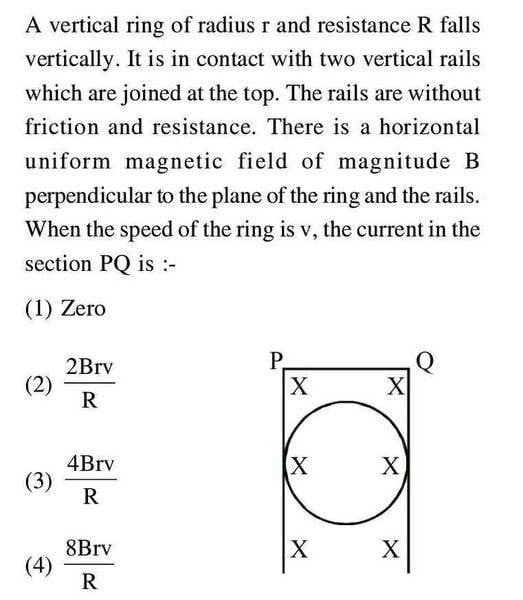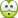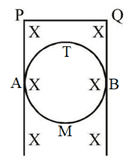# Induced EMF and current in the circuit

## Homework Statement## The Attempt at a Solution

First I will label a few points .

A is the point on the ring touching the rails , vertically below P .
B is the point on the ring touching the rails , vertically below Q .
T is the topmost point on the ring .
M is the bottommost point on the ring .

When speed of the ring is v , EMF induced between A and B will be Bv(2r) with B at higher potential. But there will be no net EMF induced in the ring as there is no change in flux .This means we can treat the two half rings as if EMF 2Bvr is induced in top half rings ATB and also EMF 2Bvr is induced in bottom half ring AMB .

Now I will write KVL in the ring in anticlockwise sense starting from A -> M -> B -> T ->A . Suppose i1 current flows through AMB and i2 current flows in ATB .Resistance of the two parts will be R/2 each .

Writing KVL in the loop of the ring , 2Bvr -i1R/2 -2Bvr + i2R/2 = 0

This gives i1 = i2 . That means current flows from A to B via M and also flows from A to B via T . It is like there are two resistances R/2 in parallel between A and B .

We also have i1=i2= 4Bvr/R .

Current flowing in PQ will be ( i1+ i2 )= 8Bvr/R from Q to P i.e option 4) . Is that correct ?

Is my work and more importantly my reasoning alright ?

#### Attachments

Last edited:

TSny
Homework Helper
Gold Member
I agree that option (4) is the correct answer.

As far as the reasoning goes, I think its OK. But, a point of contention might occur when you state
When speed of the ring is v, EMF induced between A and B will be Bv(2r) with B at higher potential.
The meaning of "EMF induced between A and B" is not really clear here, and its something that people tend to quibble about. Likewise, what does it mean to say that B is at a higher potential than A? Note that E = 0 at every point inside the conducting path BQPA since the rails have zero resistance. So, if you go from B to A along path BQPA, is there a "potential drop"?

•Jahnavi
Thank you TSny for replying .

The meaning of "EMF induced between A and B" is not really clear here, and its something that people tend to quibble about. Likewise, what does it mean to say that B is at a higher potential than A? Note that E = 0 at every point inside the conducting path BQPA since the rails have zero resistance. So, if you go from B to A along path BQPA, is there a "potential drop"?

You are right. There is no potential drop between A and B via PQ .

So , to describe the situation , would it be correct to say that end B is more positive than A just like the positive terminal of a battery ?

Another thing , if I consider PQBTA (wire and upper semicircular ring ), can I think of the semicircular arc ATB as a battery of EMF 2Bvr such that a resistanceless wire APQB is connected across this battery . It is as if this battery is shorted . There is no potential difference across the battery terminals .

Is this thinking fine ?

One more thing I would like to clarify is that when current flows from A to B via M and T , magnetic field will be generated inside the ring due to these currents ( in fact from other currents as well ), which means flux inside the ring changes . But flux should be constant .

Do we neglect this additional magnetic field inside the ring ( due to induced currents ) in comparison to external magnetic field ?

Last edited:
rude man
Homework Helper
Gold Member
Just to be a nuisance, I vote for (2). Rationale:
emf = B dA/dt
A = 2rz - πr2/2
where z is the distance from the PQ bar to the (say center) of the ring. z = z(t) and dz/dt = v.

Now for T to show me where I went wrong ...One more thing I would like to clarify is that when current flows from A to B via M and T , magnetic field will be generated inside the ring due to these currents ( in fact from other currents as well ), which means flux inside the ring changes . But flux should be constant .
Do we neglect this additional magnetic field inside the ring ( due to induced currents ) in comparison to external magnetic field ?
AsIi see it, right or wrong, there is no current in the overall ring. But suppose an isolated ring were in a time-varying B = kt (ramping up) field such that a constant current is induced. Then the answer is that the flux does change as k and thus the current are increased, and B is no longer just the externally applied field. But this problem is intractable by any reasonable math and so the assumption is implicitly made that the current generates a negligible B field of its own. Still, emf = A dB/dt still holds.

(You rigorously also have to consider the effect of the ring-induced field on the external field. That's what goes on with transformers.)

TSny
Homework Helper
Gold Member
emf = B dA/dt
A = 2rz - πr2/2
where z is the distance from the PQ bar to the (say center) of the ring. z = z(t) and dz/dt = v.
Nothing wrong here. You are considering the emf in the circuit formed by the rails and the top half of the circular ring; i.e., the circuit ATBQPA.The emf for ATBQPA is

emf = B dA/dt = B d(2rz - πr2/2)/dt = B 2rv

This emf equals the "IR-drop" around the circuit. Since the rails have zero resistance, the only IR-drop in circuit ATBQPA is along ATB. Following Jahnavi's notation, the current in ATB is i1 and the resistance in ATB is R/2 (where R is the total resistance of the circular ring). So,

i1 R/2 = B 2rv which implies that the current in ATB is i1 = 4Brv/R.

Jahnavi showed that there is an equal current i2 in the bottom half of the loop AMB. Both i1 and i2 flow from A to B, and they merge together at B to produce a total current in BQPA equal to

i1 + i2 = 8Brv/R.

#### Attachments

Last edited:
•Jahnavi
TSny
Homework Helper
Gold Member
So , to describe the situation , would it be correct to say that end B is more positive than A just like the positive terminal of a battery ?
That would be true if the ring is not touching the rails. But when the ring is making contact with the zero-resistance rails at A and B, then I don't think there will be any charge buildup at A or B. But I could be wrong here.

Another thing , if I consider PQBTA (wire and upper semicircular ring ), can I think of the semicircular arc ATB as a battery of EMF 2Bvr such that a resistanceless wire APQB is connected across this battery . It is as if this battery is shorted . There is no potential difference across the battery terminals .
I think that is an OK way to think about it. If the ring doesn't make contact with the rails as it moves downward, then A and B will become oppositely charged and they would be sort of like the terminals of a battery. But, when they are shorted by the zero-resistance rails, A and B lose their charge (I think).

One more thing I would like to clarify is that when current flows from A to B via M and T , magnetic field will be generated inside the ring due to these currents ( in fact from other currents as well ), which means flux inside the ring changes . But flux should be constant .

Do we neglect this additional magnetic field inside the ring ( due to induced currents ) in comparison to external magnetic field ?
Yes, I believe that it is generally assumed that you can ignore the B field of the induced currents in questions of this sort.

•Jahnavi
Thank you so muchrude man
Homework Helper
Gold Member
i1 + i2 = 8Brv/R.
Thanks T. I made a mistake in dividing the emf by R instead of R/2. That makes i = 4Brv/R.
Right now I don't see why there is a current in the lower half of the ring. Difficult problem, at least for me!
I am also trying to look at this from the Blv law, i.e. based on Lorentz force rather than faraday. With moving media that is supposed to be the surer-fire way to go: emf = B⋅(l x v).

Right now I don't see why there is a current in the lower half of the ring.

Net induced EMF in the ring is zeroIf there is no current in the lower half , induced EMF in the ring will be non zero .

In addition , KVL will be violated if current in lower half is zero .

Last edited:
TSny
Homework Helper
Gold Member
I am also trying to look at this from the Blv law, i.e. based on Lorentz force rather than faraday. With moving media that is supposed to be the surer-fire way to go: emf = B⋅(l x v).
Yes, if you look at it from the point of view of the Lorentz force causing movement of charge in the ring as the ring moves through the B field, then you can see why there should be current from left to right in both the top and bottom of the ring.

rude man
Homework Helper
Gold Member
OK, using T's drawing, I neglected the fact that there are two loops: APQBT and APQBM. Each generates an emf = 2BvR with current = 4Bvr/R, giving total current thru the "short" = 8Bvr/R.

Can be drawn as a schematic of a battery of emf 2Bvr in series with a resistance R/2, this in parallel with an identical battery & series resistor, then a short in parallel with both, giving short current 8Bvr/R.

And also in terms of the Bvl law: each semi-circle (arc) moves thru a constant B field, generating emf = 2Bvr (this we get by integration of emf = B⋅(dl x v) over each arc). Current in each arc flows from A to B giving total current emf/(R/2) + emf/(R/2) = 8Bvr/R. In this case the integration is simply done by replacing the arcs with separated parallel straight wires. (I prefer to use the BLv law for moving media).

So I finally agree with both of you. Sorry for the additional verbiage but I had to thrash this out for myself.#### Attachments

•TSny